# Python | Data Augmentation

Data augmentation is the process of increasing the amount and diversity of data. We do not collect new data, rather we transform the already present data. I will be talking specifically about image data augmentation in this article.
So we will look at various ways to transform and augment the image data.

1. Need for data augmentation
2. Operations in data augmentation
3. Data augmentation in Keras
4. Data augmentation using Augmentor

1. Need for data augmentation
Data augmentation is an integral process in deep learning, as in deep learning we need large amounts of data and in some cases it is not feasible to collect thousands or millions of images, so data augmentation comes to the rescue.

It helps us to increase the size of the dataset and introduce variability in the dataset.

2. Operations in data augmentation
The most commonly used operations are-

1. Rotation
2. Shearing
3. Zooming
4. Cropping
5. Flipping
6. Changing the brightness level

Now we will look at all these operations in detail. I will also provide the code for data augmentation later in the article.

The original image that I will use for illustrationRotation
Rotation operation as the name suggests, just rotates the image by a certain specified degree.
In the example below, I specified the rotation degree as 40.Shearing
Shearing is also used to transform the orientation of the image.
The result of shearing operation looks like this –Zooming
Zooming operation allows us to either zoom in or zoom out.
The result looks like this –Cropping
Cropping allows us to crop the image or select a particular area from an image.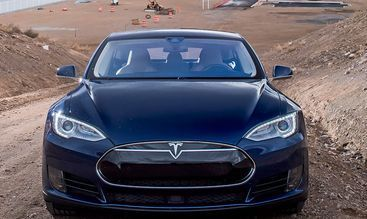Flipping
Flipping allows us to flip the orientation of the image. We can use horizontal or vertical flip.
You should use this feature carefully as there will be scenarios where this operation might not make much sense e.g. suppose you are designing a facial recognition system, then it is highly unlikely that a person will stand upside down in front of a camera, so you can avoid using the vertical flip operation.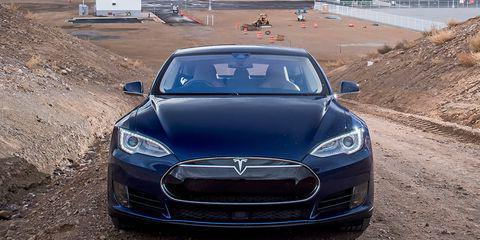Changing the brightness level
This feature helps us to combat illumination changes.You can encounter a scenario where most of your dataset comprises of images having a similar brightness level e.g. collecting the images of employees entering the office, by augmenting the images we make sure that our model is robust and is able to detect the person even in different surroundings.3. Data augmentation in Keras
Keras is a high-level machine learning framework build on top of TensorFlow. I won’t go into the details of the working of Keras, rather I just want to introduce the concept of data augmentation in Keras.
We can perform data augmentation by using the ImageDataGenerator class.
It takes in various arguments like – rotation_range, brightness_range, shear_range, zoom_range etc.

Code : Python code implementing Data augmentation

 `# Importing necessary functions ` `from` `keras.preprocessing.image ``import` `ImageDataGenerator,  ` `array_to_img, img_to_array, load_img ` `  `  `# Initialising the ImageDataGenerator class. ` `# We will pass in the augmentation parameters in the constructor. ` `datagen ``=` `ImageDataGenerator( ` `        ``rotation_range ``=` `40``, ` `        ``shear_range ``=` `0.2``, ` `        ``zoom_range ``=` `0.2``, ` `        ``horizontal_flip ``=` `True``, ` `        ``brightness_range ``=` `(``0.5``, ``1.5``)) ` `   `  `# Loading a sample image  ` `img ``=` `load_img(``'image.jpg'``)  ` `# Converting the input sample image to an array ` `x ``=` `img_to_array(img) ` `# Reshaping the input image ` `x ``=` `x.reshape((``1``, ) ``+` `x.shape)  ` `  `  `# Generating and saving 5 augmented samples  ` `# using the above defined parameters.  ` `i ``=` `0` `for` `batch ``in` `datagen.flow(x, batch_size ``=` `1``, ` `                          ``save_to_dir ``=``'preview'``,  ` `                          ``save_prefix ``=``'image'``, save_format ``=``'jpeg'``): ` `    ``i ``+``=` `1` `    ``if` `i > ``5``: ` `        ``break`

The above code snippet allows you to generate 5 augmented images having different zoom, rotation, brightness etc.

Augmented Images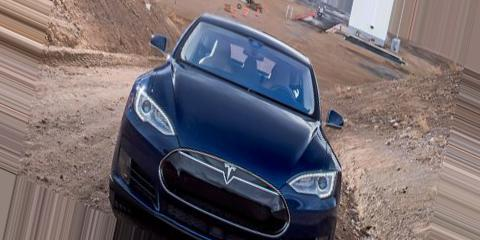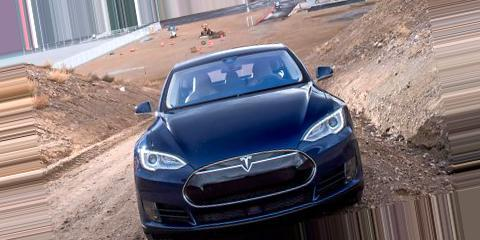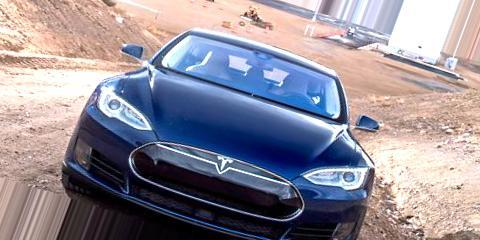4. Data augmentation using Augmentor

 `# Importing necessary library ` `import` `Augmentor ` `# Passing the path of the image directory ` `p ``=` `Augmentor.Pipeline(``"image_folder"``) ` ` `  `# Defining augmentation parameters and generating 5 samples ` `p.flip_left_right(``0.5``) ` `p.black_and_white(``0.1``) ` `p.rotate(``0.3``, ``10``, ``10``) ` `p.skew(``0.4``, ``0.5``) ` `p.zoom(probability ``=` `0.2``, min_factor ``=` `1.1``, max_factor ``=` `1.5``) ` `p.sample(``5``) `

The above code snippet allows you to generate 5 augmented images based on the features defined above.

Augmented Images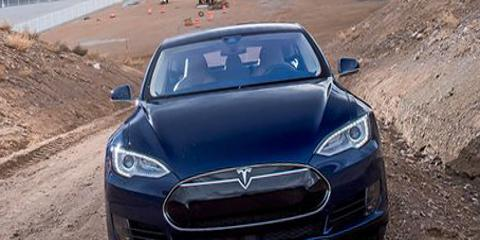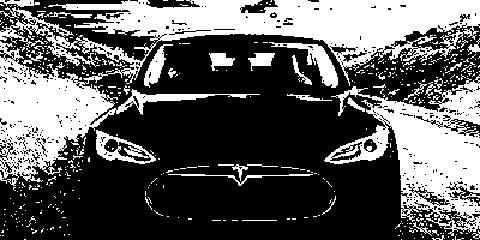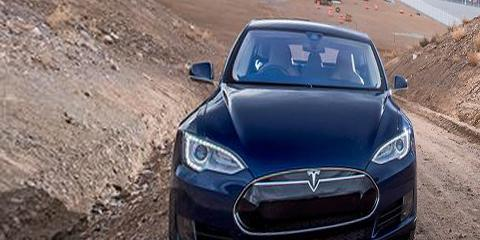References –

https://keras.io/preprocessing/image/

https://github.com/mdbloice/Augmentor

My Personal Notes arrow_drop_upCheck out this Author's contributed articles.

If you like GeeksforGeeks and would like to contribute, you can also write an article using contribute.geeksforgeeks.org or mail your article to contribute@geeksforgeeks.org. See your article appearing on the GeeksforGeeks main page and help other Geeks.

Please Improve this article if you find anything incorrect by clicking on the "Improve Article" button below.